# Adding and Subtracting Fractions With Different Denominators

Page 2 of 3
Let's do another one: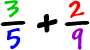The LCM of 5 and 9 is 45... So our LCD is 45.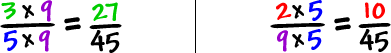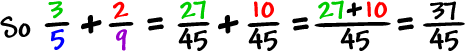Subtraction works the same way.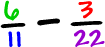The LCM of 11 and 22 is 22... So the LCD is 22.

We just need to change the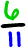.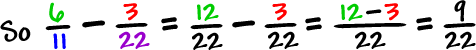Done!

Head on over to the next page to try some fraction practice problems...